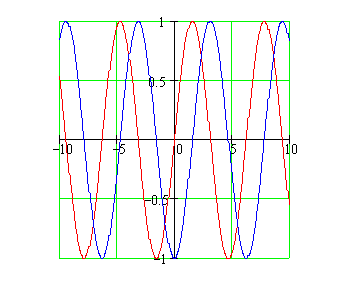Calculus on Demand at Dartmouth College Lecture 2 | Index | Lecture 4 Lecture 3## Resources

Math 3 Course Syllabus
Practice Exams

# Contents

In this lecture we review (or introduce) those aspects of the trigonometric functions that we will need for the study of calculus. The trig functions are particularly useful for modeling periodic behavior.

### Quick Question

What is the trig identity for sin(x − π/2) illustrated in the following graph?### Outline

Outline for Trigonometric Functions

### Textbook

Trigonometric Functions